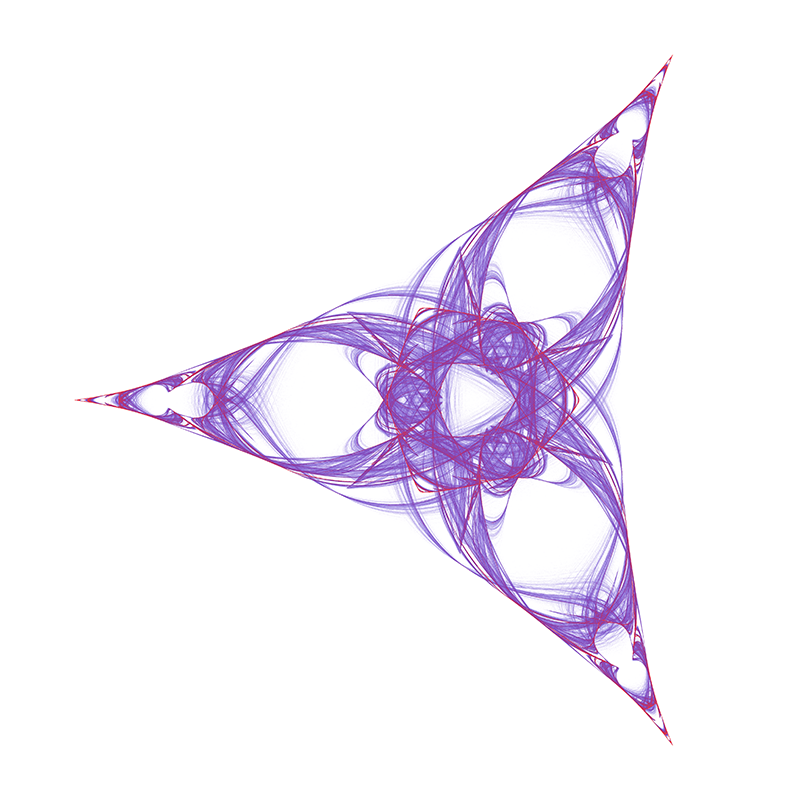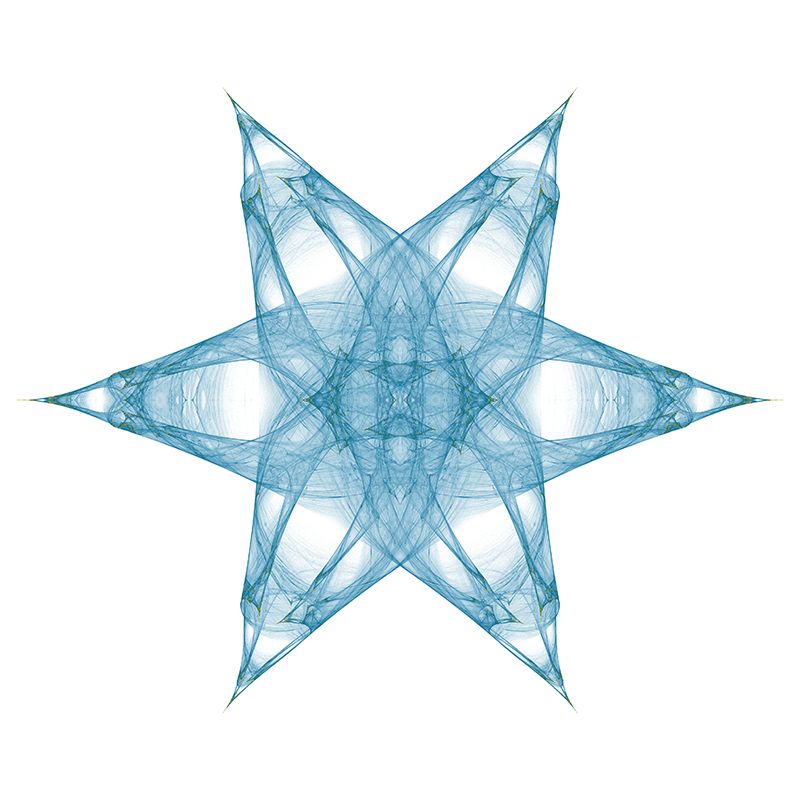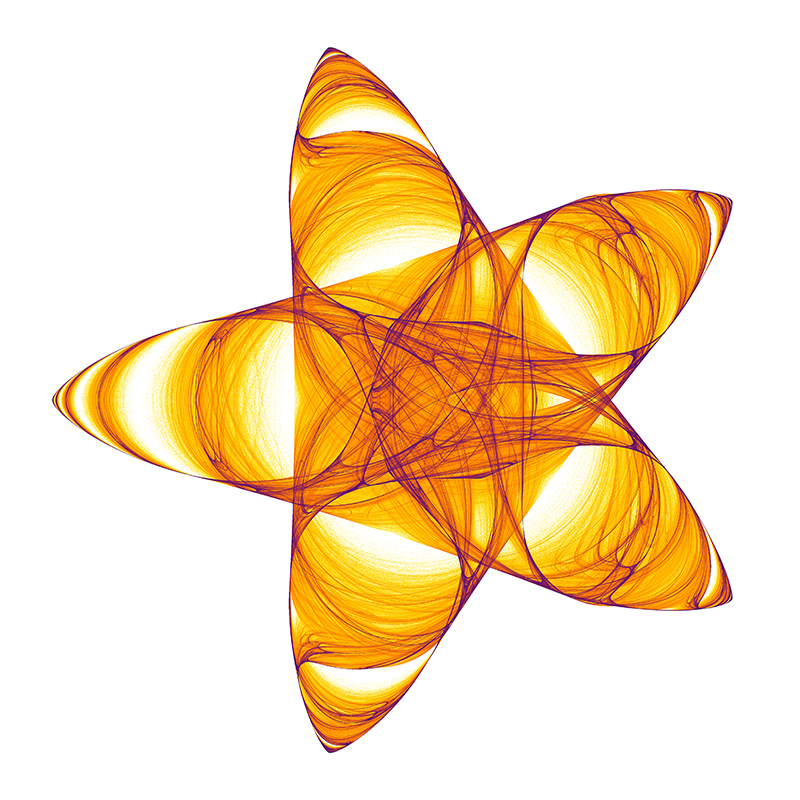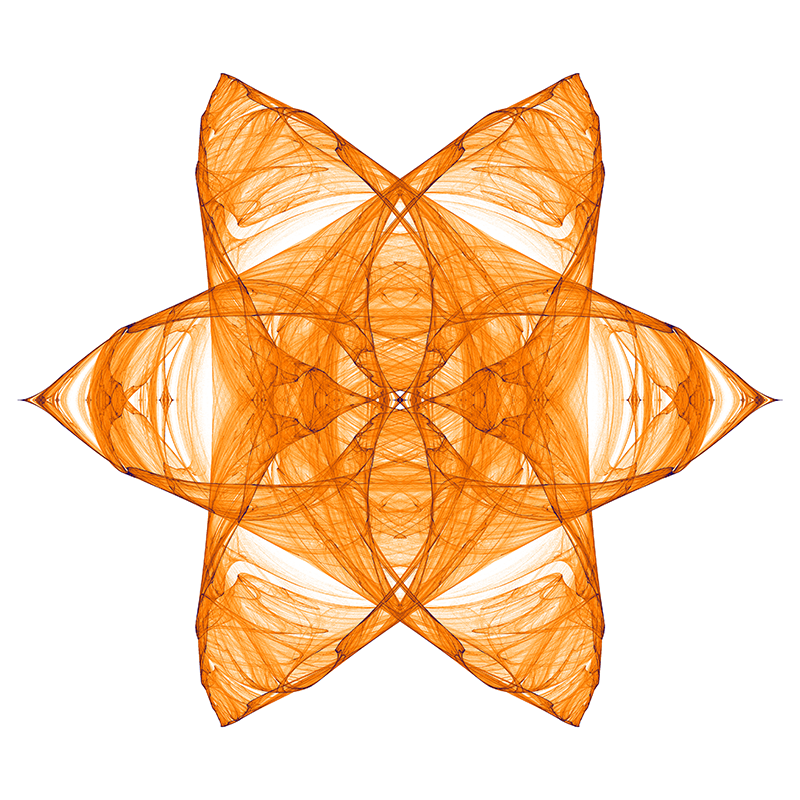# Star Julia

By Roger Bagula
C source and examples by Paul Bourke

April 2000, images updated Jan 2018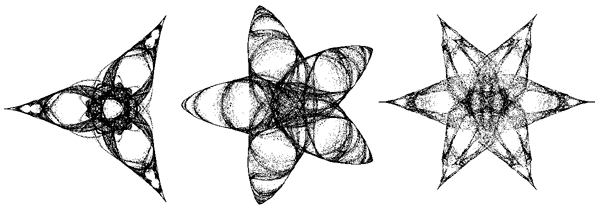This article is a result of experimentation with the reverse iteration of the fixed point Julia. In the calculation of the formula:

zc = (1 +/- sqrt(1 - 4 * c)) / 2

There are many ways you can put together the signs and there are two ones that can be made harmonic. In a series of experiments with up to four IFS transforms I got a series of stars from an experimental program by replacing one with:

zc = (exp(i * n * w) +/- sqrt(exp(i * m * w ) - 4 * c)) / 2

Where n and m are integers. I got a lot of other fractals by varying the signs, but these stars are the pretty results.